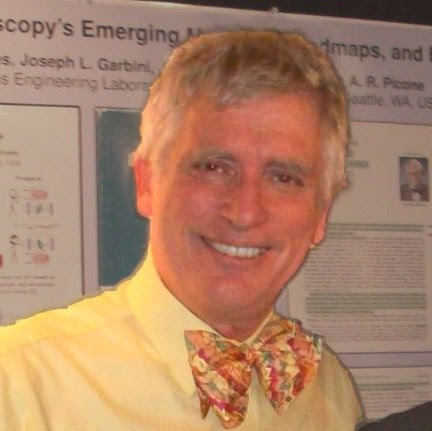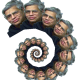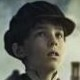Ancient mathematical objects can still contain secretsRecreational Mathematics source

David Singmaster is a mathematician who is now retired from London South Bank University, England, but he is not British—he was born in the USA. As a metagrobologist, he has a huge collection of mechanical puzzles, books on puzzles, and more. For a quick puzzle, can you name another word—of definite relevance to this blog—that ends in “-bology” (and not “-biology”)?

Today Ken and I wish to talk about Pascal’s Triangle, and a beautiful conjecture of Singmaster on the structure of the famous triangle.

We all know that Pascal’s famous triangle is formed from the binomial numbers${{n \choose k}}$. The first row is${}$, the second is${[1,1]}$, the third is${[1,2,1]}$, then comes${[1,3,3,1]}$, and so on:

The Triangle is related to the equally famous Binomial Theorem:

Theorem 1 For any${x}$ and${y}$ real numbers and${n \ge 0}$ a natural number,$\displaystyle (x + y)^{n} = \sum_{k=0}^{n} {n \choose k} x^{k} y^{n-k}.$

Whoever discovered this wonderful theorem has our thanks. One unique claimant might be Isaac Newton, who in 1665 generalized the Binomial Theorem to allow real exponents. This was a new level of sophistication that required notions of convergence, since now the sum is not finite.

A pretty use of the Binomial Theorem is a short proof of Fermat’s Little Theorem: Let${p}$ be a prime. Then by the Binomial Theorem it follows that$\displaystyle 2^{p} = (1+1)^{p} = \sum_{k=0}^{p} {p \choose k}.$

But the sum is easily shown to be congruent to${1+1 \bmod p}$. This uses that${{p \choose k} \equiv 0 \bmod p}$ for prime${p}$ and${1 < k < p}$. Thus,$\displaystyle 2^{p} \equiv 2 \bmod p.$

The general case${a^p \equiv a \bmod p}$ can be proved in the same manner.

## Who Invented This Triangle?

While Newton may be the inventor of the generalized Binomial Theorem, it far less clear who invented the Triangle and the original Binomial Theorem. Blaise Pascal is often credited with the discovery of the Triangle and the Binomial Theorem in the 1600’s. Thus we call the former Pascal’s Triangle.

As is often the case in mathematics—something we have noticed many times before—the name attached to a math concept, theorem, or object of any kind, is often not accurate. It is often just plain wrong.

A “little bit” earlier—that is, in the 4th century BCE—Euclid mentioned the special case when${n=2}$. Then the 3rd century BCE Indian mathematician Pingala included the higher powers. And it goes on:

• In the 10th century CE the Indian mathematician Halayudha and the Persian mathematician Al-Karaji knew the result.

• Also in 11th century the Persian poet and mathematician Omar Khayyam did too.

• And also in the 13th century the Chinese mathematician Yang Hui did as well.

Probably others also knew the Triangle and the theorem, and some even had proofs.

## Magic Properties of Pascal’s Triangle

There are many patterns to be found inside the Triangle. Of course the row sums are powers of${2}$, which follows directly from the Binomial Theorem. The Triangular Numbers are found in the Triangle as a diagonal:See the page “All You Ever Wanted to Know About Pascal’s Triangle and More” for many more interesting patterns, including a recurring “hockey stick” pattern.

## A Simple Conjecture

Given the Triangle has been known for hundred’s of years, perhaps even thousand of years, it is quite amazing that a simple conjecture could be found in 1971 by Singmaster. The conjecture is quite easy to state, but appears to be one of those that is going to be very hard to resolve.

Given a number${a > 1}$, let${S(a)}$ be the number of times that${a}$ occurs in the triangle. That this number is finite follows from the fact that${a}$ must occur within the first${a+1}$ rows of the triangle. Of course it occurs twice in that row, as the second and second-last entry; call these the “trivial occurrences.” Singmaster’s conjecture is:

There is an absolute constant${C}$ so that for all${a > 1}$,${S(a) \le C}$.

Paul Erdős said that Singmaster’s conjecture is “probably true,” but he suspected it would be very hard to prove. Singmaster stated the conjecture in a Math Monthly article aptly titled “How Often Does an Integer Occur as a Binomial Coefficient?” He proved that for${a>1}$,$\displaystyle S(a) = O(\log a).$

A more recent paper by Daniel Kane proves that$\displaystyle S(a) = O \left(\frac{(\log a)(\log\log\log a)}{(\log\log a)^{3}} \right).$

This is impressive, but far from the absolute constant that is conjectured. Why is this conjecture hard?

## Diofactine Analysis?

Often hardness in number theory is signalled early by weird jumps in concrete examples. For instance the Collatz “${3n+1}$problem has a suddenly long path for${n = 27}$. In Singmaster’s case the unusual small number is:$\displaystyle 3003 = \binom{78}{2} = \binom{15}{5} = \binom{14}{6}.$

Together with the two trivial occurrences this makes 8 appearances for 3003. There is an infinite family of numbers occurring 6 times, but:

No other number is known to occur 8 times. The next-highest number above 24,310 that is known to occur 6 times is

Nor is it known whether any central element${\binom{2m}{m}}$ occurs more than the statutory 3 times. Now multiple occurrences come from whole-number solutions to equations of the form$\displaystyle \binom{n}{k} = \binom{q}{\ell} = \binom{r}{m} = \dots$

The constituents of these equations are all factorials. Diophantine equations are ones required to be solved in whole numbers. We wonder if there is a particular theory of equations that must be solved in factorial numbers.

To be sure,${n}$ is recoverable as${n!/(n-1)!}$, so multiplying one side of an equation by${(n-1)!}$ while the other has${n!}$ allows one to simulate a variable${n}$ in an ordinary Diophantine set of equations. Hence an unrestricted theory of sets of factorial equations would reduce to ordinary Diophantine analysis. But perhaps an appropriate cost penalty against this trick can yield a nice theory of what we might call “Diofactine” equations.

One distinctive feature of such a theory would be reliance on analyzing the extension of factorial called the Gamma function. The proofs of the above upper bounds on${S(a)}$ exemplify this: the idea is to replace the binomial coefficient by a smooth function based on the Gamma function. Then one needs to count the number of lattice points on a certain curve. This can be pushed to yield the log-type growth of the${S}$-function. Whether it can yield a constant seems unclear.

We also find it impressive that Singmaster’s conjecture was noticed only in 1971, and not years or centuries earlier.

## Open Problems

Who really invented the Triangle? Or who discovered the Triangle? Is the conjecture correct—perhaps with${C = 8}$?

One approach that I played with and could not make work is to look at the properties of the binomial coefficients modulo primes. The famous Lucas Theorem allows us to compute the residue of${{n \choose k}}$ modulo a prime from just information about${n,k}$ modulo${p}$. I thought for a while that it might be able to at least give an elementary proof that${S(a)}$ grows slowly. But I was unable to make this work. Perhaps someone else can.

Good luck. And keep looking at the Triangle to see if there are other patterns to be discovered.

P.S.: Yesterday was the 150th anniversary of the Gettysburg Address. Since we have been discussing chalk talks versus PowerPoint recently, we can’t resist noting Peter Norvig’s hilarious sendup of how Abraham Lincoln’s presentation would have come out using PowerPoint.

[added needed qualifier “known to occur” for 6-time numbers, fixed erroneous entry 186->286 in triangle diagram (original).]

1.November 20, 2013 4:56 pm

I’m guessing you don’t mean tribology, unless there’s something about sliding planes in Dr. Who’s Triangle, so maybe symbology?

•November 20, 2013 8:46 pm

Kudos—I was thinking of you when I added that sentence to Dick’s intro. 🙂

2.November 20, 2013 6:48 pm

Diabology, very plausibly! (`cuz math is the study of devilishly hard problems).

3.November 21, 2013 7:48 am

Tuesday Newyork Times Crossword puzzle also celebrated 150th anniversary of Gettysburg address – http://rexwordpuzzle.blogspot.com/2013/11/1922-willa-cather-novel-that-won.html

4.November 21, 2013 2:14 pm

Draw the Riff and the Rote for “Four Score And Seven”.

5.November 21, 2013 9:47 pm

Herbology!

Also, I was tinkering with this conjecture for an hour or so the other day. I thought maybe I could prove a counterexample exists using the probabilistic method, but I didn’t get very far.

•November 21, 2013 9:50 pm

That’s an excellent idea: what is the asymptotic density of values of binomial coefficients, and how many “collisions” should they have…

•November 21, 2013 10:14 pm

Thanks for the vote of confidence! But once I heard that Paul Erdos thought the conjecture was true (and he must have tried to prove it using his own method!) I lost hope to prove a counterexample 😦

It seems like anything else would just be an “argument as to why the theorem should be true” and not a proof.

6.November 22, 2013 11:17 am

In reference to many previous GLL posts on quantum computing, this afternoon Gil Kalai will speak at the the University of Washington (UW) Pacific Institute for the Mathematical Science (PIMS) colloquium on the topic Why quantum computers cannot work.

This is a continuation of the wonderful Gil Kalai/Aram Harrow debate, and would be great to see quantum folks there!

As a tribute to Gil and Aram (and Scott Aaronson too), I have composed for MathOverflow a question: Do quantum “Sure-Shor separators” have a natural Veronese/Segre classification? (question inspired by Gil Kalai and Aram Harrow)

This question is constructed as a tribute to Aaronson’s fine “Sure-Shore Separator” question and the outstanding Kalai/Harrow debate that this question helped stimulate. For us engineers, proposition “Why quantum computers CAN’T work” is dual to a broad class of propositions that include “How large-scale quantum simulation DOES work” and “How atomic-resolution microscopes CAN work”, and includes too unanswered fundamental physics questions like “Is Nature’s quantum dynamical state-space EXPERIMENTALLY static and flat?”

Responses from GLL readers are invited, needless to say (and later this week I will offer a bounty on this question).

If you’re in-or-near Seattle, be sure to come to the Gil’s lecture!

7.November 25, 2013 4:26 am

Can the only way to multiply be just ‘to multiply’ or ‘repeatedly add’ (note fft techniques and other tricks come from this interpretation)? Is there another interpretation?

8.November 27, 2013 1:06 am

It is interesting that we call Pingala and Halayudha “mathematicians”. They were both actually prosodists, and their descriptions of the triangle appear in books about the structure of verses. (Halayudha’s version apparently appears in a commentary on Pingala).
A similar case is that of the Sanskrit poet Rudrata, whose description of the Hamilton cycle (at least 800 years before Hamilton) appears in a book on literary theory.

It is interesting that these “outsiders” are now almost as well known in mathematics as some of the more influential “professionals” of the age (Aryabhata, Bhaskara and Brahmagupta). Perhaps a thousand years down the line, people will be finding mathematical discoveries even in the works of Jacques Derrida. 🙂

9.•